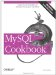# Summarizing with COUNT( )

### Summarizing with COUNT()

7.2.1 Problem

You want to count the number of rows in a table, the number of rows that match certain conditions, or the number of times that particular values occur.

7.2.2 Solution

Use the COUNT( ) function.

7.2.3 Discussion

To count the number of rows in an entire table or that match particular conditions, use the COUNT( ) function. For example, to display the contents of the records in a table, you could use a SELECT * query, but to count them instead, use SELECT COUNT(*). Without a WHERE clause, the query counts all the records in the table, such as in the following query, which shows how many rows the driver_log table contains:

```mysql> SELECT COUNT(*) FROM driver_log;
+----------+
| COUNT(*) |
+----------+
| 10 |
+----------+```

If you don't know how many U.S. states there are, this query tells you:

```mysql> SELECT COUNT(*) FROM states;
+----------+
| COUNT(*) |
+----------+
| 50 |
+----------+```

COUNT(*) with no WHERE clause is very quick for ISAM or MyISAM tables. For BDB or InnoDB tables, you may want to avoid it; the query requires a full table scan for those table types, which can be slow for large tables. If an approximate row count is all you require and you have MySQL 3.23 or later, a workaround that avoids a full scan is to use SHOW TABLE STATUS and examine the Rows value in the output. Were states an InnoDB table, the query output might look like this:

```mysql> SHOW TABLE STATUS FROM cookbook LIKE 'states'G
*************************** 1. row ***************************
Name: states
Type: InnoDB
Row_format: Dynamic
Rows: 50
Avg_row_length: 327
Data_length: 16384
Max_data_length: NULL
Index_length: 0
Data_free: 0
Auto_increment: NULL
Create_time: NULL
Update_time: NULL
Check_time: NULL
Create_options:
Comment: InnoDB free: 479232 kB```

To count only the number of rows that match certain conditions, add an appropriate WHERE clause to the query. The conditions can be arbitrary, making COUNT( ) useful for answering many kinds of questions:

• How many times did drivers travel more than 200 miles in a day?

```mysql> SELECT COUNT(*) FROM driver_log WHERE miles > 200;
+----------+
| COUNT(*) |
+----------+
| 4 |
+----------+```
• How many days did Suzi drive?

```mysql> SELECT COUNT(*) FROM driver_log WHERE name = 'Suzi';
+----------+
| COUNT(*) |
+----------+
| 2 |
+----------+```
• How many states did the United States consist of at the beginning of the 20th century?

```mysql> SELECT COUNT(*) FROM states WHERE statehood < '1900-01-01';
+----------+
| COUNT(*) |
+----------+
| 45 |
+----------+```
• How many of those states joined the Union in the 19th century?

```mysql> SELECT COUNT(*) FROM states
-> WHERE statehood BETWEEN '1800-01-01' AND '1899-12-31';
+----------+
| COUNT(*) |
+----------+
| 29 |
+----------+```

The COUNT( ) function actually has two forms. The form we've been using, COUNT(*), counts rows. The other form, COUNT(expr), takes a column name or expression argument and counts the number of non-NULL values. The following query shows how to produce both a row count for a table and a count of the number of non-NULL values in one of its columns:

`SELECT COUNT(*), COUNT(mycol) FROM mytbl;`

The fact that COUNT(expr) doesn't count NULL values is useful when producing multiple counts from the same set of values. To count the number of Saturday and Sunday trips in the driver_log table with a single query, do this:

```mysql> SELECT
-> COUNT(IF(DAYOFWEEK(trav_date)=7,1,NULL)) AS 'Saturday trips',
-> COUNT(IF(DAYOFWEEK(trav_date)=1,1,NULL)) AS 'Sunday trips'
-> FROM driver_log;
+----------------+--------------+
| Saturday trips | Sunday trips |
+----------------+--------------+
| 1 | 2 |
+----------------+--------------+```

Or to count weekend versus weekday trips, do this:

```mysql> SELECT
-> COUNT(IF(DAYOFWEEK(trav_date) IN (1,7),1,NULL)) AS 'weekend trips',
-> COUNT(IF(DAYOFWEEK(trav_date) IN (1,7),NULL,1)) AS 'weekday trips'
-> FROM driver_log;
+---------------+---------------+
| weekend trips | weekday trips |
+---------------+---------------+
| 3 | 7 |
+---------------+---------------+```

The IF( ) expressions determine, for each column value, whether or not it should be counted. If so, the expression evaluates to 1 and COUNT( ) counts it. If not, the expression evaluates to NULL and COUNT( ) ignores it. The effect is to count the number of values that satisfy the condition given as the first argument to IF( ).

The difference between COUNT(*) and COUNT(expr) is discussed further in "Summaries and NULL Values."MySQL Cookbook
ISBN: 059652708X
EAN: 2147483647
Year: 2005
Pages: 412
Authors: Paul DuBois